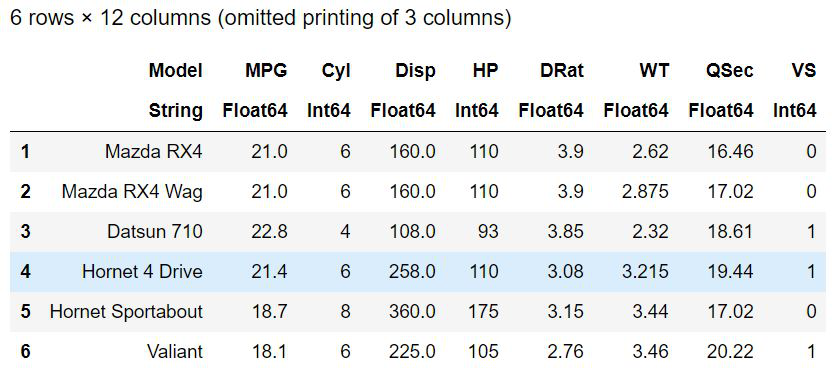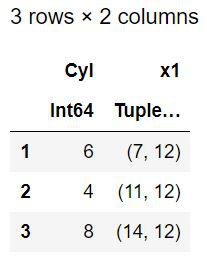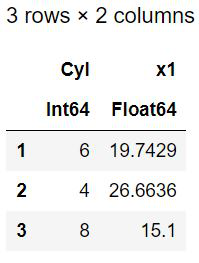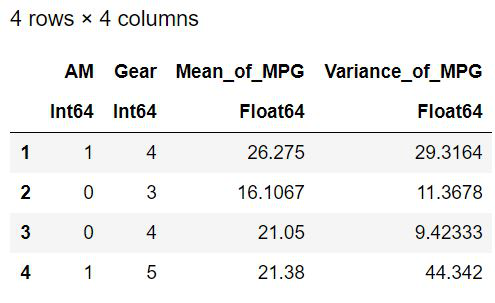Related Articles

# Data Munging in Julia

• Last Updated : 25 Aug, 2020

Data Analysis is a process using which the raw data is cleansed, transformed, and modeled in a useful way to utilize it for making decisions in business or for discovering some information. The raw data cannot be used as it is. There will be many flaws like missing values in the datasets. Moreover, the data has to be processed in some way to find information. This process of converting and mapping the raw data into some other form so that it could be effectively utilized for analysis is called data munging. It is also known as data wrangling. It is one of the most important components in data science. Some of the processes that are used for making the data useful for analysis are Split-Apply-Combine functions.

#### Packages required

The dataset can be loaded from the RDataset package. The standard datasets available in R language are made available to utilize in Julia by this package. It can be installed by writing the following command in the Julia terminal.

To load the datasets and work on multidimensional arrays like the Pandas dataframes in Python is required. Here such functionality is provided by two packages DataFrames and DataFramesMeta. These can also be installed using the Julia terminal and imported in the program. To add these packages, type the command below in the terminal.

#### Dataset Required

For illustrating the concepts of data munging, this article makes use of the dataset mtcars. It contains information of 32 automobiles of 1973-74 model. The features considered are fuel consumption and some more attributes which are listed below.

1. mpg – Miles/Gallon(US).
2. cyl – Number of cylinders.
3. disp – Displacement (cu.in).
4. hp – Gross HorsePower.
5. drat – Rear axle Ratio.
6. wt – Weight
7. qsec – 1/4 mile time
8. vs – Engine shape, where 0 denotes V-shaped and 1 denotes straight.
9. am – Transmission. 0 indicates automatic and 1 indicates manual.
10. gears – Number of forward gears
11. carb – Number of carburetors.

## Julia

 `using RDatasets``cars ``=` `dataset(``"datasets"``, ``"mtcars"``)``head(cars)`

Output:#### Using groupby() function

The groupby() function here groups the data based on the specified column. It is a function that is used to only split the dataframe. The dataset will be split into subsets if this function is used. It returns the grouped view of the subsets. The grouped dataframes can be accessed using the indices that start from 1

The syntax is:

groupby (d, :col_names, sort=false, skipmissing=false)

The parameters passed are :

• d – The dataframe.
• :col_names – The columns based on which the dataset has to be split. The column name should have a preceding colon symbol.
• sort – By default the value is false and decides whether the returned dataframe should be in sorted manner.
• skipmissing – Decides whether to skip the missing values in the dataset. Default value is false

Look at the example for better understanding.

## Julia

 `using RDatasets`` ` `# loading the dataset``cars ``=` `dataset(``"datasets"``, ``"mtcars"``)`` ` `# split based on engine type alone``groupby(cars, :VS)`

Output:

Now based on the engine type ( V-shaped or Straight) the data set is split into two. All the car models that has V-shaped engine (denoted as ‘0’) will be the first group and the cars with straight engines will be the last group. If the resultant dataframes are assigned to a variable, that also becomes a dataframe and can be accessed using index numbers. The data set can be split based on more than one column. For splitting based on two or more keys, they must be enclosed with square brackets. Look at the code snippet below:

## Julia

 `using RDatasets``cars ``=` `dataset(``"datasets"``, ``"mtcars"``)`` ` `# split based on engine type ``# and number of cylinders``res ``=` `groupby(cars, [:Cyl, :VS])`` ` `# Show the first group``res[``1``]`

Output:

│ Row │ Model                 │ MPG     │ Cyl    │ Disp      │ HP     │ DRat     │ WT       │ QSec     │ VS     │ AM    │ Gear  │ Carb  │

│         │ String                  │ Float64 │ Int64 │ Float64 │ Int64 │ Float64 │ Float64 │ Float64 │ Int64 │ Int64  │ Int64  │ Int64 │

|——-|———————|———–|——–|———-|——–|———–|———-|———–|——-|———|——–|———|

│ 1     │ Mazda RX4          │ 21.0       │ 6     │ 160.0   │ 110   │ 3.9     │ 2.62    │ 16.46   │ 0     │ 1     │ 4     │ 4     │

│ 2     │ Mazda RX4 Wag  │ 21.0       │ 6     │ 160.0   │ 110   │ 3.9     │ 2.875   │ 17.02   │ 0     │ 1     │ 4     │ 4   │

│ 3     │ Ferrari Dino         │ 19.7        │ 6     │ 145.0   │ 175   │ 3.62    │ 2.77    │ 15.5    │ 0     │ 1     │ 5     │ 6     │

Models in group 1:[“Mazda RX4”, “Mazda RX4 Wag”, “Ferrari Dino”]

#### Using by() function

It performs split-apply functions. The dataset will be split at the specified column(s) and the mentioned function will be applied to each group. The syntax is as given below:

by(d, :col_names, function, sort=false)

The parameters are:

• d – the dataframe
• :col_names – the columns based on which the data should be split.
• function – Either the built-in or user defined function to be applied on each group.
• sort – Decided whether to sort the resulting dataframe or not. Default value is false.

Example 1:  Split the dataset on the basis of number of cylinders and show the size of each group.

## Julia

 `using RDatasets``cars ``=` `dataset(``"datasets"``, ``"mtcars"``)`` ` `# splitting based on size``# and displaying eah group's size``by(cars, :Cyl, size)`

Output:The dataset is split into three groups as any car in the dataset contains either 6 cylinders or 4 cylinders or 8 cylinders. The dataframe passed to by() function is cars, the size of each group, i.e., the number of rows and columns in each group is returned as tuples. For example, Here the group-1 contains 7 rows and 12 columns.

Example 2: Split the dataset based on the number of cylinders and calculate the mean of Miles/Gallon in each group

The lambda kind of functions can also be passed as a parameter. The statistical functions for finding mean, variance, the standard deviation can be applied on appropriate columns. The function will be applied to the groups that resulted after splitting. Look at the example below. Here the dataset is split based on the number of cylinders. The mean value of the miles/gallon for each group is calculated using the mean() function for which Statistics package has to be imported.

## Julia

 `using RDatasets``using Statistics`` ` `cars ``=` `dataset(``"datasets"``, ``"mtcars"``)`` ` `# splitting based on no.of.cylinders``# Mean of the miles/gallon is calculated``by(cars, :Cyl, cars``-``>mean(cars[:MPG]))`

Output:The dataset has been split into three groups and the column X1 denotes the mean of Miles/Gallon in each group. The specified function is carried out and the resultant vector is added as a separate column.  More than one function can be carried out and a do-block syntax can also be combined with this by() function. The do-block serves as the argument for the function preceding it. For by() function the first argument is the dataframe, the next one is column names and third is the function to be carried out and the do-block serves the function’s role.

Example 3: Split the dataset based on the transmission mode and number of forward gears. Calculate the Mean and Variance of the Miles/Gallon

## Julia

 `using RDatasets``using Statistics`` ` `cars ``=` `dataset(``"datasets"``, ``"mtcars"``)`` ` `# splitting based on transmission and ``# no.of.forward gears mean and ``# variance of MPG calculation``by(cars, [:AM, :Gear]) do a``    ``DataFrame(Mean_of_MPG ``=` `mean(a[:MPG]),``              ``Variance_of_MPG ``=` `var(a[:MPG]))``end`

Output:#### Using aggregate() Function

The aggregate() function is a split-apply-combine function. It splits the dataframe based on the specified columns, applies the function gives on all the columns of the split data, and combines them as a dataframe and returns it. The syntax is given below:

aggregate ( df, :col_names, function)

• df – the dataframe.
• :col_names – the columns based on which the dataset should be split.
• function – The function to be applied on all the columns of each row. It can be either a single function or vector of functions. The functions take up vectors as arguments. These functions should return a value or vector of same length.

Look at the example below. The dataset is split based on the number of cylinders and the size of each column is returned as a single vector and combined to form a dataframe.

## Julia

 `using RDatasets`` ` `cars ``=` `dataset(``"datasets"``, ``"mtcars"``)`` ` `# Splitting based on no.of.cylinders``# display size of each column``println(aggregate(cars, :Cyl, size))`

Output:

│ Row │ Cyl   │ Model_size │ MPG_size │ Disp_size │ HP_size │ DRat_size │ WT_size │ QSec_size │ VS_size │ AM_size │ Gear_size │ Carb_size │

│         │ Int64 │ Tuple…     │ Tuple…      │ Tuple…    │ Tuple…  │ Tuple…    │ Tuple…  │ Tuple…    │ Tuple…  │ Tuple…  │ Tuple…    │ Tuple…      │

|——-|———|————-|————–|————|———–|————-|———–|————–|———-|———–|————-|————-|

│ 1   │ 6     │ (7,)       │ (7,)       │ (7,)       │ (7,)    │ (7,)        │ (7,)    │ (7,)       │ (7,)     │ (7,)     │ (7,)       │ (7,)       │

│ 2   │ 4     │ (11,)      │ (11,)    │ (11,)     │ (11,)   │ (11,)     │ (11,)   │ (11,)     │ (11,)   │ (11,)   │ (11,)     │ (11,)     │

│ 3   │ 8     │ (14,)      │ (14,)    │ (14,)     │ (14,)   │ (14,)     │ (14,)   │ (14,)     │ (14,)   │ (14,)   │ (14,)     │ (14,)     │

My Personal Notes arrow_drop_up